# discrete time system response

#### alitronic

Joined Jun 13, 2020
47
Good morning, I would like to propose my answers to the following exercise and you tell me if I am reight or not.
Exercise
We consider the cascaded LTI system of the figure 1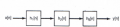Figure 1
And the impulsional response h2[n]=u[n]-u[n-2] where u[n] is a discrete Heavside signal.
The global impulsional response of the system is shwon on Figure 2.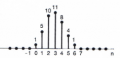Figure 2
1- Find the impulsional response h1[n].
2-Find the global response of the system if the input is x[n]=δ[n]-δ[n-1].

Since the blocks of Figure 1 are in cascade, theglobal system h[n]=h1*h2[n]*h2[n] * is the symbol of convolution
so
And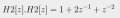From Figure 2 we can obtain the Z transform of the global response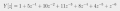From the prperties of Z transform in convolution we know that the H[z]=H1[z].H2[z].H2[z]
so H1[z]=Y[z]/(H2[z].H2[z])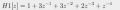And h1[n] is the discrete time signal defined by
h[n]=1 if n=0
h[n]=3 if n=1
h[n]=3 if n=2
h[n]=2 if n=3
h[n]=1 if n=4
h[n]=0 else